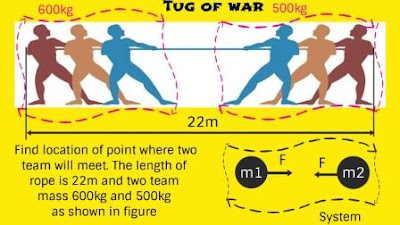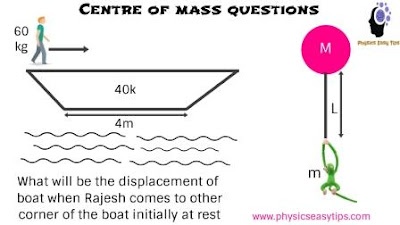centre of mass important points and numerical | Physics Easy Tips

centre of mass important points and numerical

March 31, 2018
March 31, 2018

Centre of mass important points and numerical

Today our topic is about centre of mass important points and numerical. This topic is important because we will discuss in this topic key point of centre of mass. If your theory concept is not clear you can’t solve the numerical problem. So it is very important to clear your theory concept first to solve the numerical problem.

So we are going to discuss here key theory point for center of mass and then solve the numerical problem easily.
In the previous post we have already discussed about motion of center of mass. If you have read that post i will suggest you to go through that post before learning this post.
Because we have already discussed basic and important point about centre of mass, You can refer that post motion of centre of mass.

Centre of mass always for a rigid bodies, Centre of mass is a point and the specialty of this point we suppose that whole mass of the rigid bodies lies on that point.Center of mass point may lies inside the rigid  body or  may lies outside the rigid body depends upon the shape and size of bodyThis is an imaginary point can be anywhere.If Centre of mass is taken as origin then its all particle moment or effect is zero about the origin or moment about centre of mass is always zero.ₙ∑mⱼrⱼ  = 0 this is very important result used in rotation. ₁Our laws of motion are applicable for point mass, Now in rigid body or system of particle no point mass because in system of particle every particle has mass.Now we want to apply laws of motion so we can’t directly apply laws of motion on system of particle. Hence to apply laws of motion we consider centre of mass for system of particle.Conservation of velocity of centre of mass If no external force is applied on the body then path of centre of mass is conserved.See below the question.As from the figure shown consider system of mass m1 and m2 we know that from Newton’s gravitation law two masses attract each other with equal and opposite forces as shown F on each mass m1 and m2.Now tell me what will be the net force on the system. Of course Fnet on system will be zero because forces on m1 and m2 are equal and opposite.Hence Fnet =0 so its mean that acceleration of centre of mass acom will also be zero acom =0,But tell me acceleration of m1 and m2 will be zero Big no, m1 and m2 will have some acceleration separately and moving towards each other with acceleration, don’t confuse acceleration of centre of mass of the system will be zero, because the net force   Fnet over the system is zero.  It indicate velocity of centre of mass vcom will be constant because acom is zero. Now tell me velocity of m1 and m2 will be constant Big no m1 and m2 are having acceleration hence their velocity will be variable not constant, But the velocity of center of mass will be constant.***** It is clear that if system is initially at rest then system will be finally at rest. If the system is initially in motion then system will finally in motion.This is the meaning of velocity of centre of mass constant Vcom = constant if Fnet =0 this is very very important concept.It also clear that centre of mass remain at rest drcom = 0 .Now see the below question for centre of mass.As from first boat question since given system is initially at rest when Rajesh moves from one corner to other corner. What will be displacement of boat ?Apply the concept Fnet on system is zero, acom =0 Vcom = constant but given initially at rest then Vcom = 0 , Hence centre of mass will be at rest no change.Man and Boat can move internally but centre of mass will not move will be at rest you are getting my point this is the concept.Now apply the equation for two body centre of mass.rcom  = (m₁r₁  +m₂r₂ )/(m₁+m₂)  now differentiate this equation partially we have already discussed this equation in previous post.drcom  = (m₁dr₁+m₂dr₂)/(m₁+m₂) now centre of mass at rest hence drcom = 0 put this value in above equation we will get.0 =  m₁dr₁+m₂dr₂  Now one concept here always take displacement with respect to ground .Now as from question when Rajesh will move to other corner on boat then he will travel distance 4m with respect to boat.Now suppose boat move x distance in opposite to Rajesh then actual displacement of Rajesh with respect to boat will be (4-x) = dr₁, Similarly dr₂ by boat will be ( -x ) because in negative direction.Hence put the value in above equation we will get.0  = 60(4-x) +40(-x)  or  24 – 6x -4x  = 0 10x  = 24 or x = 24/10  = 2.4m or   x = 2.4m in left direction.Now from monkey and baloon second question in above picture, Monkey is climbing on baloon rope then baloon will come down.Q By what distance the baloon shift downward when Monkey reaches at top most point neglect baloon height.Now similarly to first question here Fnet is zero and initially the system is at rest hence centre of mass will remain at rest. So we can use the equation m₁dr₁+m₂dr₂  = 0m(L-x) +M(-x) =  0  here we have suppose that baloon shift x distance downward mL -mx – Mx  =0  after simplification(m+M)x  = mLx = mL/(m+M) This is the displacement baloon will shift downward.Now we will continue in next post. I hope you have enjoyed learning centre of mass important points and numerical.  If you like comment and share thanks for reading and sharing learn and grow.Dated 7th Nov 20181.Irvin Bermers says:
•Fakhruddin Ahmad says: